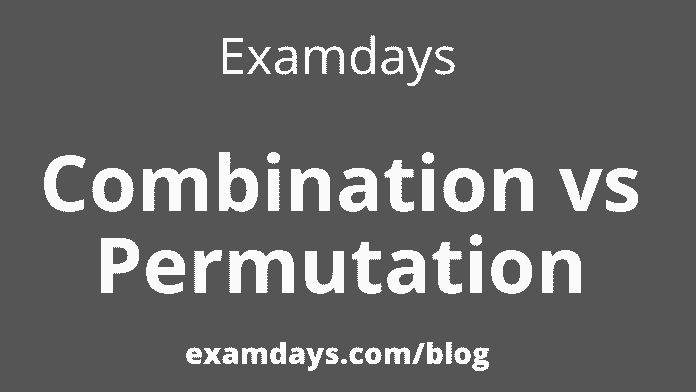# Combination vs Permutation Differences Definition Comparision for Exams

0Combination vs Permutation: The permutation is the arrangement of objects in a particular order. The sets’ members or elements are arranged in a sequence or linear order. For example, permutation 2 of set A={1,3}, for example {1,3}, {3,1}.  only the ways to arrange the elements of set A. Here is a small example to understand the concept.

When we see the schedules of trains, buses, and flights, we wonder how they are scheduled according to the convenience of people. Permutations are very helpful in preparing schedules for their departure and arrival. Also, when we see vehicle license plates with some alphabet and numbers. We can easily prepare these codes using permutations.

## Combination vs Permutation

The permutation is arranging objects in a certain way or order. Care must be taken in selection and arrangement when dealing with Permutation. In short, the order in permutations is essential. In other words, a permutation is considered an ordered combination.

Formula:

The permutation formula for n objects for a selection of r objects is given by : P(n,r) = n!/(n-r)!

For example, the number of ways of assigning 3rd and 4th positions to 10 members is given by:

P(10, 2) = 10!/(10-2)! = 10!/8! = (10.9.8!)/8! = 10 x 9 = 90.

### Types of Permutation

Permutation can be classified into three distinct categories:

->Permutation of n different objects(when repetition is not allowed).

->Repetition, where repetition is allowed.

-> Permutation when entities are not distinct(permutation of multiple sets)

Combination:

A combination is “an arrangement of objects where the order in which the objects are selected does not matter.” The combination means “selection of things,” where the order of things is unimportant. For example, if we want to buy a milkshake, we can mix any three flavors apple, banana, cherry, and durian. The combination of apple, blackberries, and cherry is the same as that of banana, apple, and cherry. So if we want to make these possible flavor combinations, Let’s look at the first letter of the fruit names and shorten them.

## Combination Formulae

A combination of 4 objects taken three times equals the number of subgroups of 3 objects taken from 4 objects. Take another example given three fruits; Say an apple, an orange, and a pear; from this set, three combinations can be taken: an apple and a pear; an apple and an orange; Or a pear and an orange.

Here are the formulae

nCk = [(n)(n-1)(n-2)….(n-k+1)]/[(k-1)(k-2)…….(1)]

which can be written as;

nCk = n!/k!(n-k)!, when n>k

nCk = 0 , when n<k

Where n = distinct object to choose from

C = Combination

K = spaces to fill (Where k can be replaced by r also)

The combination can also be represented as: –nCr, nCr, C(n,r), Crn.

## Difference Between Permutation and Combination:

What is permutation?

The permutation is the changing or arrangement of elements or objects in a linear sequence.

What is the permutation principle?

The exchange formula for n items taken r at a time is given by:
P(n,r) = n!/(n-r)!

What is the principle of permutation when repetition is allowed?

Let n be the number of objects and r be the selection of objects. Then if repetition is allowed, the permutation of objects will be n × n × n × ……(r times) = n^r.

Join Examdays Telegram

For more details about the Telegram Group, Click the Join Telegram below button.Join Membership

In case of any doubt regarding Telegram, you can mail us at [email protected].

This site uses Akismet to reduce spam. Learn how your comment data is processed.